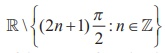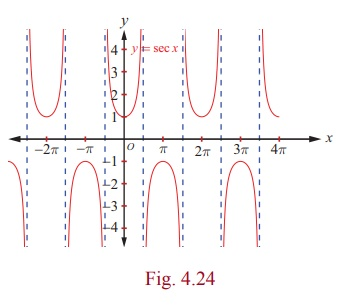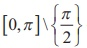Home | | Maths 12th Std | The Secant Function and Inverse Secant Function

# The Secant Function and Inverse Secant Function

The secant function is defined as the reciprocal of cosine function. So, y = sec x=1/cosx is defined for all values of x except when cos x = 0 .

The Secant Function and Inverse Secant Function

The secant function is defined as the reciprocal of cosine function. So, = sec x=1/cosx is defined for all values of except when cos = 0 .Thus, the domain of the function = sec is.

As −1 ≤ cos ≤ 1, = sec does not take values in (−1, 1) . Thus, the range of the secant function is (−∞,1] [1, ∞) . The secant function has neither maximum nor minimum. The function = sec is a periodic function with period 2π and it is also an even function.

## 1. The graph of the secant function

The graph of secant function in 0 ≤ ≤ 2 π,  x≠ π/2, 3π/2 is shown in Fig. 4.23.In the first and fourth quadrants or   in   the interval – π/2 < x < π/2, = sec x   takes   only   positive  values, whereas it takes only negative values in the second and third quadrants or in the interval π/2 < x < 3π / 2.

For 0 ≤ ≤ 2 π x ≠ π/2, 3π/2, the secant function is continuous. The value of secant function raises from 1 to ∞ for ∈ [0, π/2);  it raises from −∞ to −1 for ∈ (π/2, π]. It falls from −1 to −∞ for x [π , 3 π /2) and falls from  to 1 for x∈ (3 π/2, 2 π].As y = sec x is periodic with period 2π , the same segment of the graph for 0  ≤ x ≤ 2π , x ≠ π/2, 3 π/2, is repeated inNow, the entire graph of y = sec x is shown in Fig. 4.24.

## 2. Inverse secant function

The secant function, sec→ R \ (−1,1)  is bijective in the restricted domain.

So, the inverse secant function is defined with R \ (−1,1) as its domain and withas its range.

### Definition 4.7

The secant function, sec-1 : R\(-1, 1) → [0,π ] \ {π/2} is defined by sec-1 (x) = y whenever sec y = x and y [0,π ] \ {π/2 } .

## 3. Graph of the inverse secant function

The inverse secant function, = sec−1 is a function whose domain is R \ (−1,1) and the range is

[ - π/2, π/2]\{0}. That is, cosec-1 : (-∞, -1] U [1, ∞) → [ - π/2, 0) U (0, π/2].

Fig. 4.25 and Fig. 4.26 are the graphs of the secant function in the principal domain and the inverse secant function in the corresponding domain, respectively.### Remark

A nice way to draw the graph of = sec or cosec :

(i) Draw the graph of = cos or sin

(ii) Draw the vertical asymptotes at the -intercepts and take reciprocals of values

Tags : Definition, Graph, Properties , 12th Mathematics : UNIT 4 : Inverse Trigonometric Functions
Study Material, Lecturing Notes, Assignment, Reference, Wiki description explanation, brief detail
12th Mathematics : UNIT 4 : Inverse Trigonometric Functions : The Secant Function and Inverse Secant Function | Definition, Graph, Properties